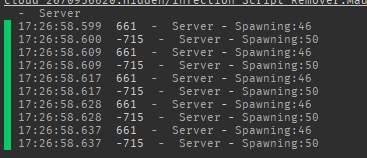# math.Random not so random anymoreSo I’ve had a script which randomly selects an enemy based off of a random number and decides a random place to insert said enemy.Now for some weird reason, the math.random part of the script seems to not be working anymore (it generates the same random number for the chosen enemy and the same random number for the chosen location (X and Z)Here’s the full script:

``````wait (5)

local spawns = script.Parent.Spawns:GetChildren()
local maxCount = 100 -- how many enemies to generate in total
local count = 0

RunService = game:GetService("RunService")

if RunService:IsStudio() then -- makes it easier on your computer if you test script in studio

maxCount = 5

end

local Spawntime = 1

local Name = script.Parent.Name
local Templates = game.ServerStorage:WaitForChild("TemplateEnemies")
local Area = Templates:WaitForChild(Name)

for _, child in ipairs(spawns) do

child.Transparency = 1

end

while count < maxCount do -- The problems are localized in this part:

local randomspawn = spawns[math.random(1, #spawns)]

local spawnmaxX = randomspawn.Position.X + (randomspawn.Size.X / 2)
local spawnminX = randomspawn.Position.X - (randomspawn.Size.X / 2)

local spawnmaxZ = randomspawn.Position.Z + (randomspawn.Size.Z / 2)
local spawnminZ = randomspawn.Position.Z - (randomspawn.Size.Z / 2)

local spawnY = randomspawn.Position.Y

local AreaTemplates = Templates:WaitForChild(Name)
local selection = math.random(1, #AreaTemplates:GetChildren())

local toclone = Templates:WaitForChild(Name):WaitForChild(selection)

local ranx = math.random(spawnminX, spawnmaxX)

print (ranx)

local ranz = math.random(spawnminZ, spawnmaxZ)

print (ranz)

local Clone = toclone:Clone()
Clone.Parent = script.Parent
Clone:SetPrimaryPartCFrame(CFrame.new(ranx, spawnY, ranz))

count = count + 1

end
``````And this is what the output of the positioning looks like:My question is: how could I make it so that the math.Random is actually random

2 Likes
``````local Players = game:GetService("Players")
local Player = Players.LocalPlayer

local RandomObject = Random.new(tick())
local RandomNumber = RandomObject:NextInteger(1, Player.UserId)
print(RandomNumber)
``````

Consider using the “Random” class instead.

https://developer.roblox.com/en-us/api-reference/datatype/Random

The provided code snippet prints a random integer between 1 and the local player’s user ID inclusively.

4 Likes

You can set the random seed to tick() at the start of your script.

``````math.randomseed(tick())
``````

For whatever reason a lot of times studio will pick the same random seed for the start which will result in the same “randomness” exactly.

Though to be honest I would do what Forummer said using the random class instead.

3 Likes